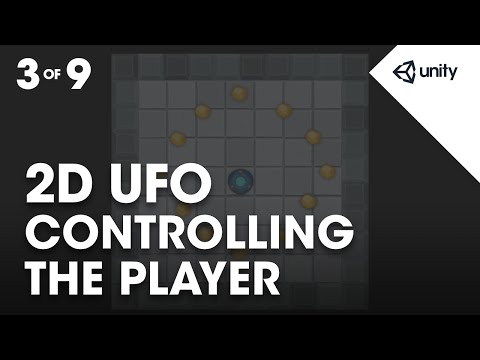# Controlling the Player

#### Difficulty: Beginner

In this assignment we'll add a Rigidbody 2D to our player so that they can moved using physics and write a simple C# script to allow us to move the player around the play field.## Controlling the Player

Beginner 2D UFO tutorial

Transcripts

### Code snippet

``````using UnityEngine;
using System.Collections;

public class CompletePlayerController : MonoBehaviour {

public float speed;             //Floating point variable to store the player's movement speed.

private Rigidbody2D rb2d;       //Store a reference to the Rigidbody2D component required to use 2D Physics.

// Use this for initialization
void Start()
{
//Get and store a reference to the Rigidbody2D component so that we can access it.
rb2d = GetComponent<Rigidbody2D> ();
}

//FixedUpdate is called at a fixed interval and is independent of frame rate. Put physics code here.
void FixedUpdate()
{
//Store the current horizontal input in the float moveHorizontal.
float moveHorizontal = Input.GetAxis ("Horizontal");

//Store the current vertical input in the float moveVertical.
float moveVertical = Input.GetAxis ("Vertical");

//Use the two store floats to create a new Vector2 variable movement.
Vector2 movement = new Vector2 (moveHorizontal, moveVertical);

//Call the AddForce function of our Rigidbody2D rb2d supplying movement multiplied by speed to move our player.
rb2d.AddForce (movement * speed);
}
}``````

#### Related tutorials#### 2D UFO tutorial

1. Controlling the Player